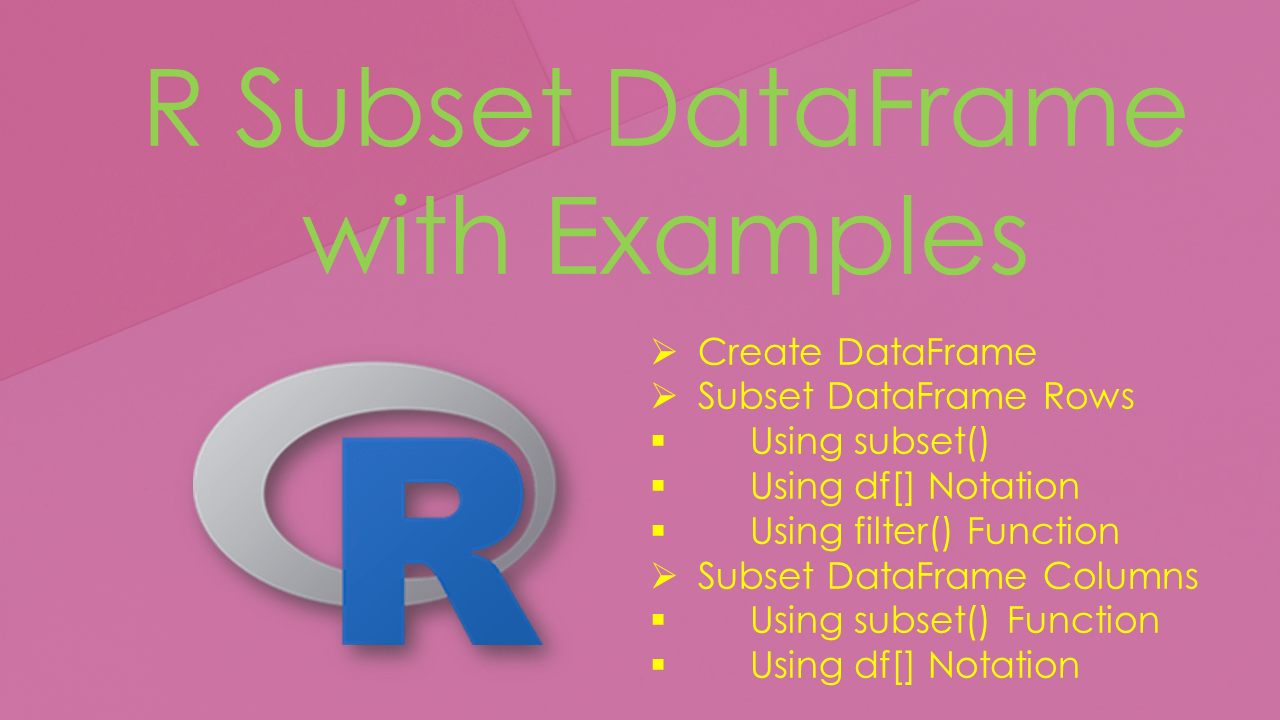# Subset Data Frame in R with Examples

If you wanted to get the subset of a data.frame (DataFrame) Rows & Columns in R, either use the subset() function , `filter()` from `dplyr` package or R base square bracket notation `df[]`. subset() is a generic R function that is used to get the rows and columns (In R terms observations & variables) from the data frame. Also used to get a subset of vectors, and subset of matrices.

In this article, I will explain different ways to subsetting the R DataFrame rows and columns. Alternatively, you can also select rows in R using df[] notation.

## 1. Create DataFrame

Let’s create a DataFrame in R, run the examples to subset data.frame (DataFrame) rows and columns. and explore the output.

``````
# Create DataFrame
df <- data.frame(
id = c(10,11,12,13,14,15,16,17),
name = c('sai','ram','deepika','sahithi','kumar','scott','Don','Lin'),
gender = c('M','M',NA,'F','M','M','M','F'),
dob = as.Date(c('1990-10-02','1981-3-24','1987-6-14','1985-8-16',
'1995-03-02','1991-6-21','1986-3-24','1990-8-26')),
state = c('CA','NY',NA,NA,'DC','DW','AZ','PH'),
row.names=c('r1','r2','r3','r4','r5','r6','r7','r8')
)
df
``````

Yields below output.

``````
id    name gender        dob state
r1 10     sai      M 1990-10-02    CA
r2 11     ram      M 1981-03-24    NY
r3 12 deepika   <NA> 1987-06-14  <NA>
r4 13 sahithi      F 1985-08-16  <NA>
r5 14   kumar      M 1995-03-02    DC
r6 15   scott      M 1991-06-21    DW
r7 16     Don      M 1986-03-24    AZ
r8 17     Lin      F 1990-08-26    PH
``````

## 2. Subset DataFrame Rows

subset() is used to get the observations and variables from the data frame (DataFrame). Also used to get a subset of vectors, and a subset of matrices.

### 2.1 Using subset()

This function takes a syntax `subset(x, subset, select, drop = FALSE, …)` where the first argument is the input object, the second argument is the subset expression and the third is to specify what variables to select.

``````
# subset by row name
subset(df, subset=rownames(df) == 'r1')

# subset row by vector of row names
subset(df, rownames(df) %in% c('r1','r2','r3'))

# subset by condition
subset(df, gender == 'M')

# subset by condition with %in%
subset(df, state %in% c('CA','DC'))

# subset by multiple conditions using |
subset(df, gender == 'M' | state == 'PH')

# subset by multiple conditions using &
subset(df, gender == 'M' & state %in% c('CA','NY'))
``````

### 2.1 Using df[] Notation

By using bracket notation on R data.frame we can subset rows by name, by index, by column, and by condition e.t.c

``````
# Select Rows by Index
df[3,]

# Select Rows by List of Index Values
df[c(3,4,6),]

# Select Rows by Index Range
df[3:6,]

# Select Rows by column value
df[df\$gender == 'M',]

# Select Rows by vector of Values
df[df\$state %in% c('CA','AZ','PH'),]

# Select Rows by Checking multiple conditions
df[df\$gender == 'M' & df\$id > 15,]
``````

### 2.3 Using filter() Function

Similarly, you can also subset the data.frame by using `filter()` function from `dplyr` package. In order to use this, you have to install it first using `install.packages('dplyr')` and load it using `library(dplyr)`.

``````
# Using dplyr::filter
dplyr::filter(df, state %in% c("CA", "AZ", "PH"))
``````

## 3. Subset DataFrame Columns

In this section, I will cover how to subset DataFrame (data.frame) columns by using the subset() method, `df[]` notation, and `filter()` from `dplyr` package.

### 3.1 Using subset() Function

The below examples subset’s DataFrame (data.frame) columns by name and index.

``````
#subset columns by Name
subset(df,gender=='M',select=c('id','name','gender'))

#subset columns by Index
subset(df,gender=='M',select=c(1,2,3))
``````

### 3.2 Using df[] Notation

By using df[] notation you can also subset the columns. From the following, the example gets the columns with indices 2 and 3 and the second gets the same result but uses the column names.

``````
# Select columns with indices 2 & 3
df[,c(2,3)]

# Selects columns name and gender
df[,c('name','gender')]
``````

## 4. Complete Example of R Subset Data Frame

``````
# Create DataFrame
df <- data.frame(
id = c(10,11,12,13,14,15,16,17),
name = c('sai','ram','deepika','sahithi','kumar','scott','Don','Lin'),
gender = c('M','M',NA,'F','M','M','M','F'),
dob = as.Date(c('1990-10-02','1981-3-24','1987-6-14','1985-8-16',
'1995-03-02','1991-6-21','1986-3-24','1990-8-26')),
state = c('CA','NY',NA,NA,'DC','DW','AZ','PH'),
row.names=c('r1','r2','r3','r4','r5','r6','r7','r8')
)
df

# subset by row name
subset(df, subset=rownames(df) == 'r1')

# subset row by vector of row names
subset(df, rownames(df) %in% c('r1','r2','r3'))

# subset by condition
subset(df, gender == 'M')

# subset by condition with %in%
subset(df, state %in% c('CA','DC'))

# subset by multiple conditions using |
subset(df, gender == 'M' | state == 'PH')

# subset by multiple conditions using &
subset(df, gender == 'M' & state %in% c('CA','NY'))

# subset Rows by Index
df[3,]

# subset Rows by List of Index Values
df[c(3,4,6),]

# subset Rows by Index Range
df[3:6,]

# subset Rows by column value
df[df\$gender == 'M',]

# subset Rows by vector of Values
df[df\$state %in% c('CA','AZ','PH'),]

# subset Rows by Checking multiple conditions
df[df\$gender == 'M' & df\$id > 15,]

# Using dplyr::filter
dplyr::filter(df, state %in% c("CA", "AZ", "PH"))

# Subset columns by Name
subset(df,gender=='M',select=c('id','name','gender'))

# subset columns by Index
subset(df,gender=='M',select=c(1,2,3))

# subset columns with indices 2 & 3
df[,c(2,3)]

# subset columns name and gender
df[,c('name','gender')]
``````

## 5. Conclusion

In this article, you have learned how to Subset the data frame rows and columns in R by using the `subset()` function, `filter()` from `dplyr` package, and using `df[]` notation.

## References

### Naveen (NNK)

Naveen (NNK) is a Data Engineer with 20+ years of experience in transforming data into actionable insights. Over the years, He has honed his expertise in designing, implementing, and maintaining data pipelines with frameworks like Apache Spark, PySpark, Pandas, R, Hive and Machine Learning. Naveen journey in the field of data engineering has been a continuous learning, innovation, and a strong commitment to data integrity. In this blog, he shares his experiences with the data as he come across. Follow Naveen @ @ LinkedIn